# Signals of the Oscillator Stochastic

This module of signals is based on the market models of the oscillator Stochastic. The mechanism of making trade decisions based on signals obtained from the modules is described in a separate section.

## Conditions of Generation of Signals

Below you can find the description of conditions when the module passes a signal to an Expert Advisor.

Signal Type

Description of Conditions

• Reverse — the oscillator turned upwards (the oscillator rises at the analyzed bar and falls at the previous one).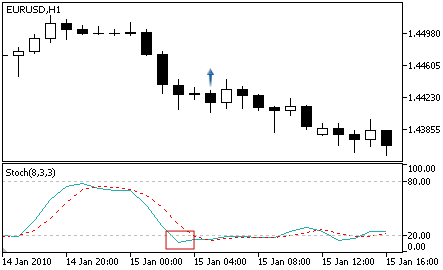• Crossing of the main and signal line — the main line is above the signal line at the analyzed bar and below the signal line at the previous one.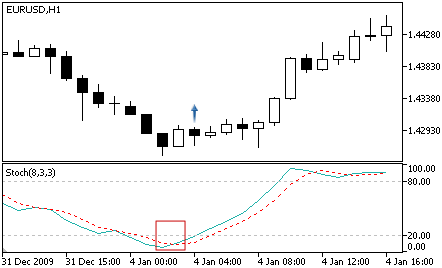• Divergence — the first analyzed bottom of the oscillator is higher than the previous one, and the corresponding price bottom is lower than the previous one.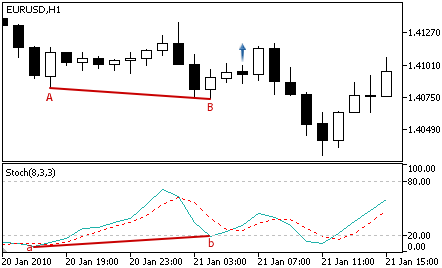• Double divergence — the oscillator form three consequent bottoms, each of them is higher than the previous one; and the price formed three corresponding bottoms, and each of them is lower than the previous one.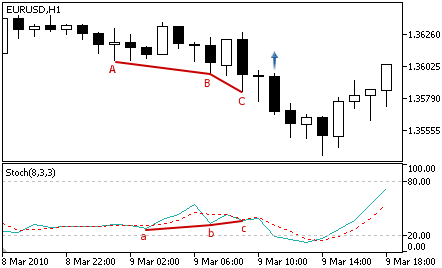For selling

• Reverse — the oscillator turned downwards (the oscillator falls at the analyzed bar and rises at the previous one).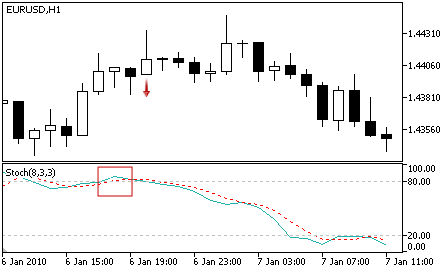• Crossing of the main and signal line — the main line is below the signal line at the analyzed bar and above the signal line at the previous one.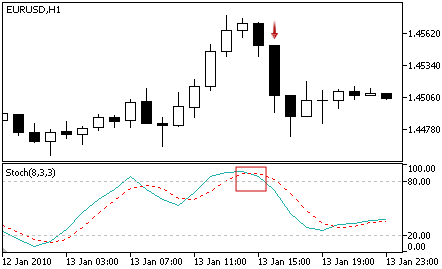• Divergence — the first analyzed peak of the oscillator is lower than the previous one, and the corresponding price peak is higher than the previous peak.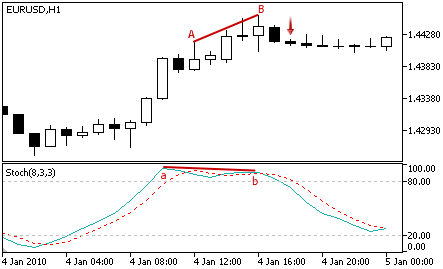• Double divergence — the oscillator formed three consequent peaks, each of them is lower than the previous one; and the price formed three corresponding peaks, each of them is higher than the previous one.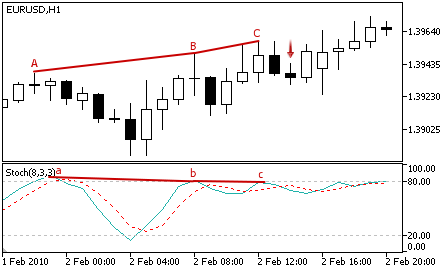Value of the oscillator grows at the analyzed bar.

No objections to selling

Value of the oscillator falls at the analyzed bar.

Note

Depending on the mode of operation of an Expert Advisor ("Every tick" or "Open prices only") an analyzed bar is either the current bar (with index 0), or the last formed bar (with index 1).

This module has the following adjustable parameters:

Parameter

Description

Weight

Weight of signal of the module in the interval 0 to 1.

PeriodK

Period of calculation of the main line of the oscillator.

PeriodD

Period of averaging of the main line of the oscillator.

PeriodSlow

Period of slowing.

Applied

A price series used for calculation of the oscillator.